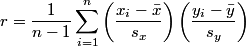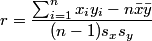# How to calculate correlation accurately

Pearson’s correlation coefficient r is used to measure the linear correlation of one set of data with another. It also provides an example of how you can get in trouble if you just take a formula from a statistics book and naively turn it into a program. I will take two algebraically equivalent equations for the correlation coefficient commonly found in textbooks and give an example where one leads to a correct result and the other leads to an absurd result.Lets take a look at two expressions for the correlation coefficient, both commonly given in textbooks.

Expression 1:Expression 2:The two expressions for r are algebraically equivalent. However, they can give very different results when implemented in software.

To demonstrate the problem, I first generated two vectors each filled with 1000 standard normal random samples. Both expressions gave correlation 0.0626881. Next I shifted my original samples by adding 100,000,000 to each element. This does not change the correlation, and the program based on the first expression returned 0.0626881 exactly as before. However, the program based on the second expression returned -8.12857.

Not only is a correlation of -8.12857 inaccurate, it’s nonsensical because correlation is always between -1 and 1.

What went wrong? The second expression for r computes a small number as the difference of two very large numbers. The two terms in the numerator are each around 1020 and yet their difference is around 0.06. That means that if calculated to infinite precision, the two terms would agree to 21 significant figures. But a floating point number (technically a double in C) only has 15 or 16 significant figures. That means the subtraction cannot be carried out with any precision on a typical computer.

Don’t draw the conclusion that the second expression is accurate unless it completely fails. The same phenomena that caused a complete loss of accuracy in this example could cause a partial loss of accuracy in another example. The latter could be worse. For example, we might not have suspected a problem if the software had returned 0.10 when the correct value was 0.06.

The same problem comes up over and over again in statistics, such as when computing sample variance or simple regression coefficients. In each case, there are two commonly used formulas, and the formula easier to apply manually is potentially inaccurate. To make matters worse, books sometimes imply that the more accurate formula is only for theoretical use and that the less accurate formula is preferable for computation.

For a more detailed explanation of why the two expressions for correlation coefficient gave such different results when implemented in software, see Theoretical explanation of numerical results.

## 8 thoughts on “How to calculate correlation accurately”

1. Karl Ove Hufthammer

Thanks for posting this. I enjoy reading about numerical issues in statistical computing. It’s surprising to many that calculating statistics from such simple formulas could be problematic.

Even something as simple as calculating the sum or average of a set of numbers can be done in numerical ‘not so smart’ ways. For instance, sorting the values before adding them (and then adding the smaller numbers before the larger ones) should give more accurate results.

I tried looking at source code for R, to find out how it calculated averages, and if it used this method. If I remember correctly (and read the code correctly), it first adds all the numbers and divides the sum by n to get a ‘naïve’ average, and then adds the average difference between the numbers and this average (which mathematically of course should be zero) to get a final value. Seems very clever.

Do you know of any (easy to read, suitable for bedtime reading) books (or papers) on numerical calculations specifically for statistics?

2. One of my favorite books on numerical computing is Numerical methods that work by Forman S. Acton. It’s not specifically about statistics, and it’s a little dated, but it’s great on the fundamentals.

3. Dear Karl and John,

I could not completely understand the problem in computation of averages. Can you please guide to understand these kinds of problems that arise in various computaional algorithm.

Regards,

Gautam

4. flashman

Dear Mr.John

What will happen if (sx==0) or (sy==0) in the first expression.
Example I want to calculate the correlation of two arrays, and with the first array, all elements are equal. Could I use the first expression.

Thanks!

5. If sx is zero, all your x’s are the same. If sy is zero, all your y’s are the same. In either case, you have a degenerate situation. I suppose you could say the correlation is zero since a constant is independent of any random variable, but correlation coefficient implies there’s a bivariate normal model in the background and that assumption is violated if one of the components is constant.

6. flashman